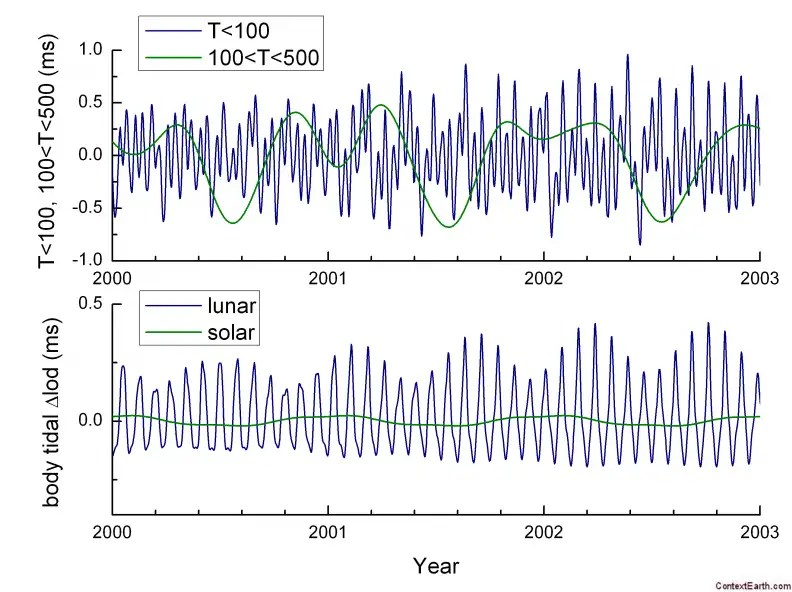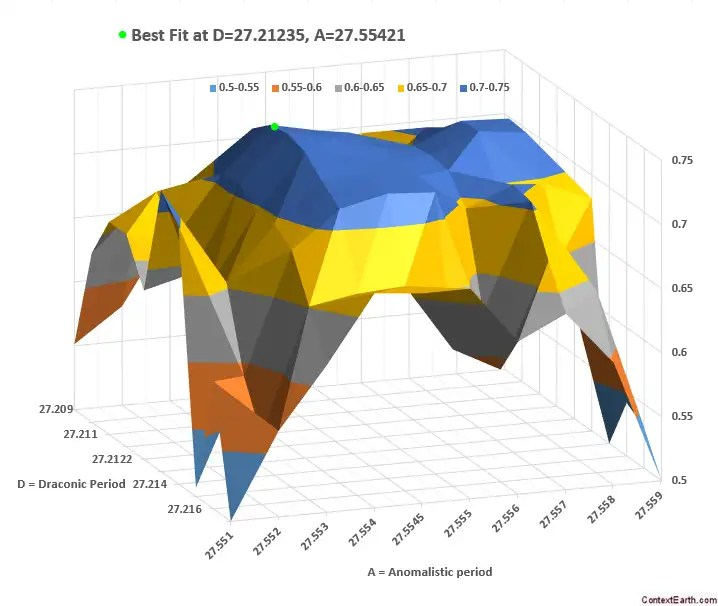# ENSO+QBO Elevator Pitch

Most papers on climate science take pages and pages of exposition before they try to make any kind of point. The excessive verbiage exists to rationalize their limited understanding of the physics, typically by explaining how complex it all is.

Conversely, think how easy it is to explain sunrise and sunset. From a deterministic point of view  and from our understanding of a rotating earth and an illuminating sun, it’s trivial to explain that a sunrise and sunset will happen once each per day. That and perhaps another sentence would be all that would be necessary to write a research paper on the topic …  if it wasn’t already common knowledge. Any padding to this would be unnecessary to the basic understanding. For example, going further and explaining why the earth rotates amounts to answering the wrong question. Thus the topic is essentially an elevator pitch.

If sunset/sunrise is too elementary an example, one could explain ocean tides. This is a bit more advanced because the causal connection is not visible to the eye. Yet all that is needed here is to explain the pull of gravity and the orbital rate of the moon with respect to the earth, and the earth to the sun. A precise correlation between the lunisolar cycles is then applied to verify causality. One could add another paragraph to explain how mixed tidal effects occur, but that should be enough for an expository paper.

We could also be at such a point in our understanding with respect to ENSO and QBO. Most of the past exposition was lengthy because the causal factors could not be easily isolated or were rationalized as random or chaotic. Yet, if we take as a premise that the behavior was governed by the same orbital factors as what governs the ocean tides, we can make quick work of an explanation.

The basic premise is that ENSO is a long-period sloshing of the Pacific ocean’s thermocline which is forced by slight changes to the earth’s rotation rate. This is analogous to the tidal forcing described above but is of a much longer period because the inertial response of the ocean is much slower than the tidal response.  The essential idea is that the change in the earth’s rotation results in the situation whereby the ocean will want to keep moving due to Newton’s first law. This change is significant — about 1 millisecond over the span of a few weeks as shown in Figure 1 below — and has an even greater impact in the reduced gravity environment of a thermocline .Fig 1:  From . The upper BLUE curve shows the measured change in the length-of-day in milliseconds due to periods of less than 100 days, which corresponds to long-period tidal cycles. The lower blue curve shows the expected tidal influence from the lunar orbit. A one millisecond change in LOD over the span of two weeks will translate the volume of the Pacific by 0.5 meters due to inertia.

That gives the qualitative and order-of-magnitude rationale for how ENSO sloshing can be initiated. Next, comes the mathematical formulation.

There is a simple, almost toy, model for ENSO that assumes actions are delayed in time. In the most basic instance, the delay is from the previous year and this feedback is placed into the context of a delay differential equation.   The idea here is that the response builds up over the course of a year and may be released the following year as a feedback — this generates a biennial see-saw which has long been hypothesized. But to make this biennial feedback sharp and temporally distinct, the forcing is accentuated at a specific time of the year, likely synchronized with the strongest solar signal.

So, with that information as a premise, we can apply the lunar forcing from the LOD measurements (of Figure 1) as an input to the ENSO model. By amplifying the response in Nov/Dec of each year, the model response matches the ENSO time-series, as shown in Figure 2 below.Fig 2: The cyclic lunar forcing as detected from LOD measurements (lower) fed into a model of ENSO (upper).   The model is trained or calibrated from 1880 to 1980, and validated beyond 1980.

This is a sharp model fit. The two cycles that form the LOD time-series correspond to highly distinct Draconic and Anomalistic periods as shown in Figure 3.  Any values even slightly away from the precise monthly values quickly degrade the model fit correlation.Fig 3:  Slightly varying the known Draconic and Anomalistic periods from their known values results in a rapidly degrading correlation.

That is exactly what happens with tidal analysis as well — it is well known that the lunar periods match with a high degree of precision to the cycles of sea-level height tide measurements.  This relationship is so solid that it is often used to rule out causality to lunar forcing should the correlation not exist.  By the same token, Richard Lindzen has on more than one occasion stressed the idea that if tidal periods are detected in the observational data then that is the likely forcing as well.This concise explanation with appropriate modifications also applies to QBO.

Now consider that the situation with ENSO is that in all the pages of research content written on the subject, no one has produced a compelling enough physical model that it can be used to fit the data at all.   It would be unbelievable if after all these years we didn’t have a physically-plausible model for ocean tides. The underlying pattern could not have remained hidden for this long. For ENSO, it’s just unfortunate that the lunar pattern was not as transparently obvious as it was for the tidal cycles.

## References

  Laplace considered the stochastic aspect in terms of the Sunrise problem, or “What is the probability that the sun will rise tomorrow?”.   This illustrates the difficulty of using probability theory when evaluating the plausibility of statements or beliefs.

 Na, Sung-Ho, et al. “Characteristics of Perturbations in Recent Length of Day and Polar Motion.” Journal of Astronomy and Space Sciences 30 (2013): 33-41.

 Gent, Peter R., and Mark A. Cane. “A reduced gravity, primitive equation model of the upper equatorial ocean.” Journal of Computational Physics 81.2 (1989): 444-480.

## 6 thoughts on “ENSO+QBO Elevator Pitch”

1.admin

What happens if the earth suddenly stops rotating

This is the extreme case, way beyond the subtle effect needed in this model

Like

2. Pingback: Confirmation Bias | context/Earth